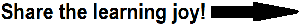Grade 1 Math: Geometry WorksheetsGrade 1 Math: Geometry
 www.studenthandouts.com > First Grade > First Grade Math > Geometry

CCSS.MATH.CONTENT.1.G.A.1 - Distinguish between defining attributes (e.g., triangles are closed and three-sided) versus non-defining attributes (e.g., color, orientation, overall size); build and draw shapes to possess defining attributes.

CCSS.MATH.CONTENT.1.G.A.2 - Compose two-dimensional shapes (rectangles, squares, trapezoids, triangles, half-circles, and quarter-circles) or three-dimensional shapes (cubes, right rectangular prisms, right circular cones, and right circular cylinders) to create a composite shape, and compose new shapes from the composite shape.

CCSS.MATH.CONTENT.1.G.A.3 - Partition circles and rectangles into two and four equal shares, describe the shares using the words halves, fourths, and quarters, and use the phrases half of, fourth of, and quarter of. Describe the whole as two of, or four of the shares. Understand for these examples that decomposing into more equal shares creates smaller shares.